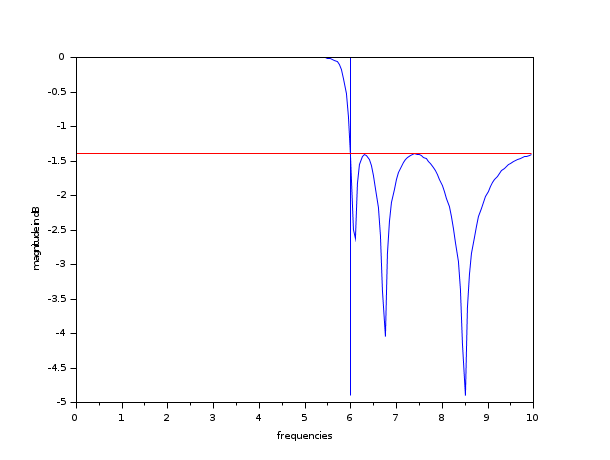Scilab Home page | Wiki | Bug tracker | Forge | Mailing list archives | ATOMS | File exchange
Change language to: English - Français - Português - 日本語 -

Справка Scilab >> Signal Processing > filters > cheb2mag

# cheb2mag

response of type 2 Chebyshev filter

### Calling Sequence

`[h2]=cheb2mag(n,omegar,A,sample)`

### Arguments

n

integer ; filter order

omegar

real scalar : cut-off frequency

A

attenuation in stop band

sample

vector of frequencies where cheb2mag is evaluated

h2

vector of Chebyshev II filter values at sample points

### Description

Square magnitude response of a type 2 Chebyshev filter.

`omegar` = stopband edge, `sample` = vector of frequencies where the square magnitude `h2` is desired.

### Examples

```//Chebyshev; ripple in the stopband
n=10;
omegar=6;
A=1/0.2;
Samples=0.0001:0.05:10;
h2=cheb2mag(n,omegar,A,Samples);
plot(Samples,log(h2)/log(10))
xtitle("", "frequencies", "magnitude in dB");

//Plotting of frequency edges
minval=(-max(-log(h2)))/log(10);
plot2d([omegar;omegar],[minval;0],,"000");

//Computation of the attenuation in dB at the stopband edge
attenuation=-log(A*A)/log(10);
plot2d(Samples',attenuation*ones(Samples)',,"000")```